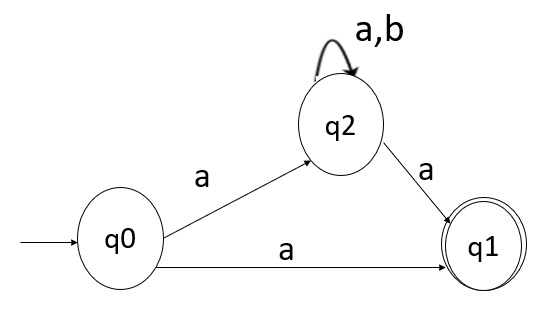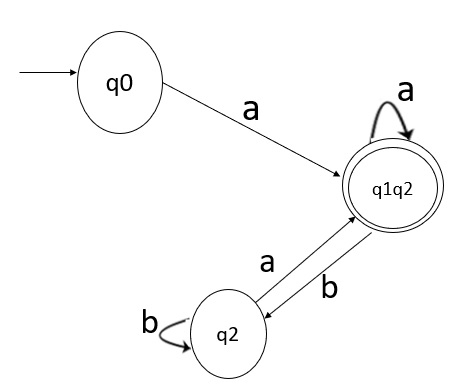# Convert NFA to DFA and explain the difference between them

Some basic difference between a Non-deterministic finite automata (NFA) and Deterministic Finite Automata (DFA) is that in DFA each state has an output for each of the inputs, whereas this is not necessary for NFA.

Also, in NFA the input can be \epsilon but in DFA this is not the case.

Moreover, in NFA one state can go to a different state on the same output but this is not the case with DFA.

Usually, we convert an NFA into a DFA by making the state transition diagram for the given NFA and accordingly vary that state diagram for the DFA. From this state transition diagram, we can make the DFA.

## Problem

Convert the given NFA to DFA.

## Solution

The given NFA is as follows −The transition table for NFA is as follows

State\inputab
->q0{q2,q1}-
q1--
q2{q1,q2}-

The transition table for DFA is as follows

State\inputab
->q0[q0q1]-
*[q2q1][q1q2][q2]
[q2][q2q1][q2]

The DFA transition diagram is as follows −